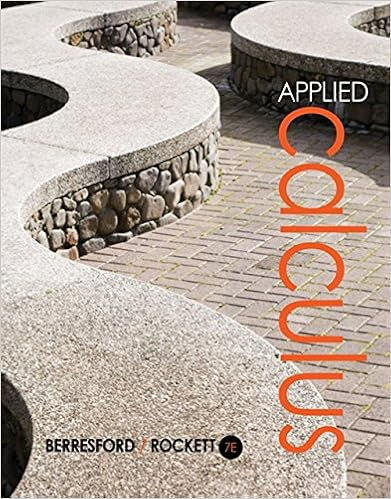# E on thanksgiving day 2012 the new england patriots

• Notes
• 244
• 75% (4) 3 out of 4 people found this document helpful

This preview shows page 123 - 128 out of 244 pages.

##### We have textbook solutions for you!
The document you are viewing contains questions related to this textbook.The document you are viewing contains questions related to this textbook.
Chapter 8 / Exercise 32
Applied Calculus
Berresford/RockettExpert Verified
e. On Thanksgiving Day, 2012, the New England Patriots scored 35 points in the second quarter to aid in their eventual 49 - 19 victory over the New York Jets. What was their unit rate of scoring per minute during that quarter (15 minutes)? If they had continued the second half (two more quarters) at that rate, what would the final score have been (New England scored 0 points in the first quarter).
##### We have textbook solutions for you!
The document you are viewing contains questions related to this textbook.The document you are viewing contains questions related to this textbook.
Chapter 8 / Exercise 32
Applied Calculus
Berresford/RockettExpert Verified
Basic Arithmetic Lesson 7 – Ratios, Rates & Proportions118
Basic Arithmetic Lesson 7 – Ratios, Rates & Proportions119 LESSON 7 – ASSESS YOUR LEARNING Work the following to assess your learning of the concepts in this lesson. Try to write complete solutions and show as much work as you can. Answers are in the back. 1. Write each ratio in simplest form. a. 5 to 10 b. 8:14 c.feetfeet462. Write each rate in simplest form. a. 60 miles in 2 hours b. 36 inches to 3 feet c. 14 men to 21 women 3. Write the unit rate for each of the following. Round to the nearest hundredth. a. 180 miles in 3 hours b. \$25.00 for 6 gallons c. 86 pounds per 16 square inches 4. Solve each of the following proportion problems for the given variable. Round to two decimals as needed. a. 1254=xb. 7215=rc. 32810x=5. Solve each of the following proportion problems for the given variable. Round to two decimals as needed. a. 839=xb. 835=rc. x28107=
Basic Arithmetic Lesson 7 – Ratios, Rates & Proportions120 6. Solve each of the following proportion problems for the given variable. Round to two decimals as needed. a. 12841x=b. 8215.3t=c. 87.6=x7. Solve the following problems using the 5-step problem solving process. Include the categories: Given, Goal, Math work, Check and Final result as a complete sentence. a. Ray can bike 25 miles in 3 hours. At this rate, how long will it take him to bike 43 miles? b. Julia can feed 8 relatives with a 12 pound turkey. How many pounds of turkey does she need to feed 14 relatives?
Basic Arithmetic Lesson 8 – Statistics121 LESSON 8 - STATISTICS INTRODUCTION In this lesson, we will learn the basic language and concepts related to a branch of mathematics that deals with collecting, organizing, and interpreting data. This branch of mathematics is called statistics. In addition, the word statisticsis often used to denote the data and information that are being collected and interpreted. The table below shows the specific objectives that are the achievement goal for this lesson. Read through them carefully now to gain initial exposure to the terms and concept names for the lesson. Refer back to the list at the end of the lesson to see if you can perform each objective. Lesson Objective Related Examples Define and compute three different measures of central tendency1, YT2 Define and compute weighted averages3, 4, YT5 Discuss data variabilityand compute the range of a data set 6, 7, YT8 Compute measures of central tendencywith data sets that contain outliers9 Use tables and graphsto interpret and analyze data 10, 11, YT12, 13, YT14 KEY TERMS The key terms listed below will help you keep track of important mathematical words and
•••